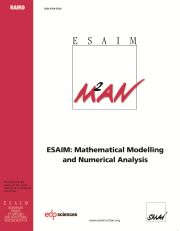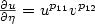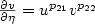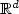Home
Hostname: page-component-684899dbb8-p6h7k Total loading time: 0.849 Render date: 2022-05-25T12:10:07.504Z Has data issue: true Feature Flags: { "shouldUseShareProductTool": true, "shouldUseHypothesis": true, "isUnsiloEnabled": true, "useRatesEcommerce": false, "useNewApi": true }ESAIM: Mathematical Modelling and Numerical Analysis

# Simultaneous vs. non-simultaneous blow-up in numerical approximations of aparabolic system with non-linear boundary conditions

Published online by Cambridge University Press:  15 April 2002

## Abstract

We study the asymptotic behavior of a semi-discrete numerical approximation for a pair of heat equations ut = Δu, vt = Δv in Ω x (0,T); fully coupled by the boundary conditions $\frac{\partial u}{\partial\eta} = u^{p_{11}}v^{p_{12}}$, $\frac{\partial v}{\partial\eta} = u^{p_{21}}v^{p_{22}}$on ∂Ω x (0,T), where Ω is a bounded smooth domain in ${\mathbb{R}}^d$. We focus in the existence or not of non-simultaneous blow-up for a semi-discrete approximation (U,V). We prove that if U blows up in finite time then V can fail to blow up if and only if p11 > 1 and p21 < 2(p11 - 1) , which is the same condition as the one for non-simultaneous blow-up in the continuous problem. Moreover, we find that if the continuous problem has non-simultaneous blow-up then the same is true for the discrete one. We also prove some results about the convergence of the scheme and the convergence of the blow-up times.

Type
Research Article
Information

## Access options

Get access to the full version of this content by using one of the access options below. (Log in options will check for institutional or personal access. Content may require purchase if you do not have access.)

## References

Abia, L.M., Lopez-Marcos, J.C. and Martinez, J., Blow-up for semidiscretizations of reaction diffusion equations. Appl. Numer. Math. 20 (1996) 145-156. CrossRef
L.M. Abia, J.C. Lopez-Marcos and J. Martinez, On the blow-up time convergence of semidiscretizations of reaction diffusion equations. Appl. Numer. Math. 26 (1998) 399-414.
G. Acosta, J. Fernández Bonder, P. Groisman and J.D. Rossi. Numerical approximation of a parabolic problem with nonlinear boundary condition in several space dimensions. Preprint.
Amann, H., Parabolic evolution equations and nonlinear boundary conditions, J. Differential Equations. 72 (1988) 201-269. CrossRef
Bandle, C. and Brunner, H., Blow-up in diffusion equations: a survey. J. Comput. Appl. Math. 97 (1998) 3-22. CrossRef
Berger, M. and Kohn, R.V., A rescaling algorithm for the numerical calculation of blowing up solution. Comm. Pure Appl. Math. 41 (1988) 841-863. CrossRef
Budd, C.J., Huang, W. and Russell, R.D., Moving mesh methods for problems with blow-up. SIAM J. Sci. Comput. 17 (1996) 305-327. CrossRef
Chen, Y.G., Asymptotic behaviours of blowing up solutions for finite difference analogue of $u_t = u_{xx} + u^{1+ \alpha}$. J. Fac. Sci. Univ. Tokyo Sect. IA Math. 33 (1986) 541-574.
P.G. Ciarlet, The Finite Element Method for Elliptic Problems. North-Holland Publishing Company, Amsterdam, New York, Oxford (1978).
Durán, R.G., Etcheverry, J.I. and Rossi, J.D., Numerical approximation of a parabolic problem with a nonlinear boundary condition. Discrete Contin. Dyn. Syst. 4 (1998) 497-506.
Elliot, C.M. and Stuart, A.M., Global dynamics of discrete semilinear parabolic equations. SIAM J. Numer. Anal. 30 (1993) 1622-1663. CrossRef
Fernández Bonder, J. and Rossi, J.D., Blow-up vs. spurious steady solutions. Proc. Amer. Math. Soc. 129 (2001) 139-144. CrossRef
A.R. Humphries, D.A. Jones and A.M. Stuart, Approximation of dissipative partial differential equations over long time intervals, in D.F. Griffiths et al., Eds., Numerical Analysis 1993. Proc. 15th Dundee Biennal Conf. on Numerical Analysis, June 29-July 2nd, 1993, University of Dundee, UK, in Pitman Res. Notes Math. Ser. 303, Longman Scientific & Technical, Harlow (1994) 180-207.
C.V. Pao, Nonlinear Parabolic and Elliptic Equations. Plenum Press, New York (1992).
Pinasco, J.P. and Rossi, J.D., Simultaneousvs. non-simultaneous blow-up. N. Z. J. Math. 29 (2000) 55-59.
Rossi, J.D., On existence and nonexistence in the large for an N-dimensional system of heat equations with nontrivial coupling at the boundary. N. Z. J. Math. 26 (1997) 275-285.
A. Samarski, V.A. Galaktionov, S.P. Kurdyunov and A.P. Mikailov, Blow-up in QuasiLinear Parabolic Equations, in Walter de Gruyter, Ed., de Gruyter Expositions in Mathematics 19, Berlin (1995).
A.M. Stuart and A.R. Humphries, Dynamical systems and numerical analysis, in Cambridge Monographs on Applied and Computational Mathematics 2, Cambridge University Press, Cambridge (1998).

# Save article to Kindle

Note you can select to save to either the @free.kindle.com or @kindle.com variations. ‘@free.kindle.com’ emails are free but can only be saved to your device when it is connected to wi-fi. ‘@kindle.com’ emails can be delivered even when you are not connected to wi-fi, but note that service fees apply.

Find out more about the Kindle Personal Document Service.

Simultaneous vs. non-simultaneous blow-up in numerical approximations of a parabolic system with non-linear boundary conditions
Available formats
×

# Save article to Dropbox

To save this article to your Dropbox account, please select one or more formats and confirm that you agree to abide by our usage policies. If this is the first time you used this feature, you will be asked to authorise Cambridge Core to connect with your Dropbox account. Find out more about saving content to Dropbox.

Simultaneous vs. non-simultaneous blow-up in numerical approximations of a parabolic system with non-linear boundary conditions
Available formats
×

# Save article to Google Drive

To save this article to your Google Drive account, please select one or more formats and confirm that you agree to abide by our usage policies. If this is the first time you used this feature, you will be asked to authorise Cambridge Core to connect with your Google Drive account. Find out more about saving content to Google Drive.

Simultaneous vs. non-simultaneous blow-up in numerical approximations of a parabolic system with non-linear boundary conditions
Available formats
×
×